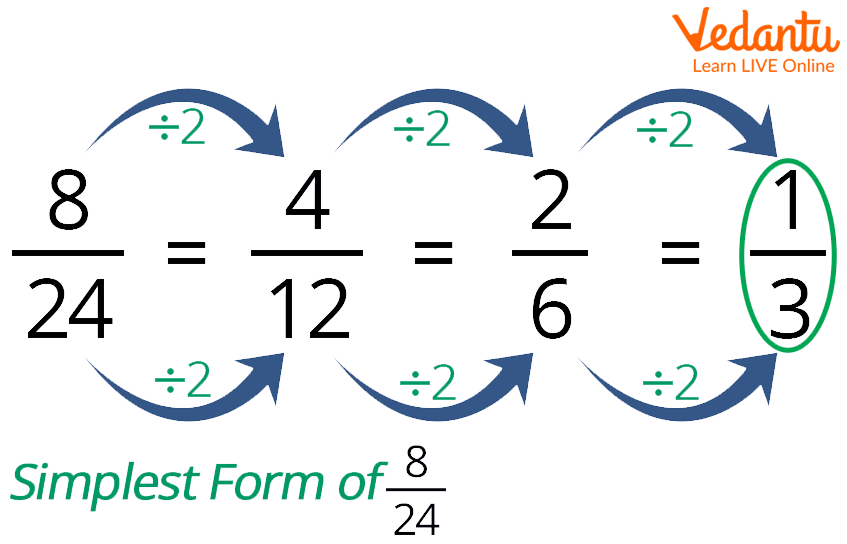Courses
Courses for Kids
Free study material
Free LIVE classes
More

# Reducing FractionsLIVE
Join Vedantu’s FREE Mastercalss

## Define Reduced Fractions:

The simplest form of a fraction is one with a reasonably prime denominator and numerator. It indicates that, except from 1, there is no common factor between the fraction's numerator (upper portion or top) and denominator (lower part or bottom).

## How to Find the Lowest Form of Fractions?

Any fraction can be easily found in its simplest form. By dividing both the numerator and denominator by the greatest common factor that entirely divides them, we need to simplify the fraction's numerator and denominator. Both the numerator and the denominator should still be whole numbers after division. Reducing fractions is another name for this process of simplifying fractions.

Since the common factor "c" is subtracted from both the numerator and the denominator, the simplified form of a fraction like "$\dfrac{{ac}}{{bc}}$ " will be "$\dfrac{a}{b}$ ".Showing Simplest Form of $\dfrac{8}{{24}}$

## Steps to find Lowest Form:

• Find the fraction's GCF for the numerator and denominator.

• By the generated GCF, divide the numerator and denominator.

• Write the given fraction's reduced fraction.

## Simple Form Ratio:

A ratio is denoted by the ratio $a:b$, where a and b are whole numbers. When we express a ratio in its simplest form, we are simplifying it down to its most basic components.

## Reduce the Fraction to Lowest Term Illustration:

Let's use the fractions $\dfrac{5}{9}$  and $\dfrac{6}{9}$  as an illustration to help you comprehend. $\dfrac{5}{9}$ is expressed in the simplest terms possible because there is just one element that connects 5 and 9. However, $\dfrac{6}{9}$ is not since 6 and 9 share a factor of 3.

Divide both the numerator and the denominator by the highest common factor, in this case 3 (since both are divisible by 3), to express 69 in the simplest words possible:

$\dfrac{{6 \div 3}}{{9 \div 3}}$

$\dfrac{6}{9}$ is $\dfrac{2}{3}$ when expressed in the simplest terms.Simplest Form for 6 / 9

These are also referred to as reducing fractions.

## Conclusion:

A fraction's simplest form is one with a denominator and numerator that are both suitably prime numbers. It shows that the numerator (upper section or top) and denominator of the fraction do not share any factors, with the exception of one. Now we will solve  non terminating recurring numbers examples.

## Solved Examples:

Example 1: Simplify it: $32:40$ .

The same approach that we used to simplify the fraction can also be used to simplify the ratio.

Here, 32 acts as the antecedent, and 40 acts as the consequent. In order to obtain the simplified form, we must discover the highest factor that can be formed from 32 and 40.

Thus, the common factor between 32 and 40 is 8.

As a result, 4:5 is the simplest version of 32:40 (because$8 \times 4 = 32{\rm{ and }}8 \times 5 = 40$)

Example 2: Reduce the fraction to its simplest form: 2:10.

We can therefore write $\dfrac{2}{{10}} = \dfrac{1}{5}$

The result of dividing the numerator and denominator by two is  $\dfrac{2}{{10}} = \dfrac{1}{5}$.

Therefore, $\dfrac{1}{5}$ is the answer.

Example 3: Are the lowest and highest forms equivalent?

If q is positive, the integers p and q have no common factor other than 1, and the rational number's lowest form allows for a possible negative value, the rational number is said to be in its standard form.

Last updated date: 03rd Oct 2023
Total views: 68.4k
Views today: 0.68k

## FAQs on Reducing Fractions

1. What is the lowest term of 8 / 10?

$\dfrac{8}{{10}} = \dfrac{{8 \div 2}}{{10 \div 2}} = \dfrac{4}{5}$ .

2. What does fractional standard form mean?

When the numerator and denominator of a fraction are both co-prime numbers, the fraction is said to be in standard form.

3. Can I get a response with a negative denominator?

It doesn't matter if the negative sign appears before the denominator, the numerator, or the entire fraction as long as you only use one.

4. Write the simplest form of 1 : 1 .

It's already in its simplest form.

Thus, the simplest form of 1:1 is 1:1.

5. How can I calculate $\dfrac{{30}}{{70}}$ in its simplest form?

The largest common divisor of 30 and 70 must be identified in order to determine the fraction's simplest form, which is $\dfrac{{30}}{{70}}$. GCD is 10 among 30 and 70. To obtain the simplest version, divide the numerator and denominator by 10. Hence,$\dfrac{{30}}{{70}} = \dfrac{{30 \div 10}}{{70 \div 10}} = \dfrac{3}{7}$ .